## LARGE FUNCTION

The LARGE function returns the nth-largest value within a collection. The largest value is ranked number 1.

The Syntax of the function is

LARGE(data, rank_c)

data:  A collection of values. data may be a number,date,duration,set is a collection containing number, date, or duration values. All values must be of the same type.

rank_c:  A number representing the size ranking of the value you want to retrieve. ranking is a number value and must be in the range of 1 to the number of values in the collection.

Notes:

A ranking of 1 retrieves the largest number in the collection, 2 the second-largest, and so on. Values included in the array that are of the same size are ranked together, but impact the outcome.

We will try to understand the function with an example.

The Sample data set is as follows:if we want to identify the large three numbers of above data set  conventional way of writing the formula is as follows: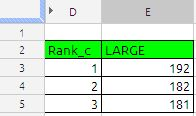The formula in cell E3 is

=LARGE(\$B\$2:\$B\$12,D3) and drag it down till E5 we will get the 1st largest 2nd largest and 3rd largest.

other way to extract largest 3 value with more robust formula is with use of ROWS function. the formula will be

=LARGE(\$B\$2:\$B\$11,ROWS(E\$3:E3)) and drag it down here we do not need a helped column D where argument rank_c.

Google Docs way of writing the formula is

=ArrayFormula(if(len(D3:D5);large(B2:B12,D3:D5);iferror(1/0)))

In the above formula you do not require to drag any thing the formula will automatically put 1st, 2nd and 3rd largest value in cell E3 to E5.

if we want to largest numbers across the columns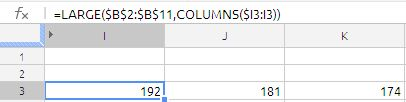The formula in cell I3 is

=LARGE(\$B\$2:\$B\$11,COLUMNS(\$I3:I3)) just drag it across.

if we want the sum of large three numbers in single cell,  formula will be as follows:

=sum(large(B2:B12;{1,2,3}))

which is nothing but sum of  192+182+181 = 555.

## IRR Function

IRR (Internal rate of return)

In simple financial terms the IRR means: The rate at which the net cash inflow are equal to net cash outflows.

The IRR function returns the internal rate of return for an investment that is based on a series of potentially irregular cash flows (payments that do not need to be a constant amount that occur at regular time intervals.

Syntax of the formula is:

IRR(values, guess)

Values: A collection that contains the cash flow values. values is a collection containing number values. Income (a cash inflow) is specified as a positive number, and an expenditure (a cash outflow) is specified as a negative number. There must be at least one positive and one negative value included within the collection. Cash flows must be specified in chronological order and equally spaced in time (for example, each month). If a period does not have a cash flow, use 0 for that period.

guess:  An optional argument specifying the initial estimate for the rate of return. guess is a number value and is either entered as a decimal (for example, 0.1) or with a percent sign (for example, 10%). If omitted, 10% is assumed. If the default value does not result in a solution, initially try a larger positive value. If this does not result in an outcome, try a small negative value.

remember this is a optional argument, even if you leave this argument results will  not get effected.

Notes:

(1) Values : Range  of cells with cash flows. Cash out is negative, cash in is positive.       Ranges of values must contain at least one positive and one negative value.

(2) Guess is not required. But if you get a #NUM! error, try different guesss – once you think might be close.

(3) Cash flow must happen at the end of each period.

(4) Cash flow start at time 0

(5) Cash flow do not have to be equal in amount.

(6) Time between each cash flow must be the same.

(7) IRR gives you the period rate. If you give it annual cash flows, it gives you annual rate, if you want a monthly rate multiply the result rate with 12 ( this is the secret).

Now lets see an example

.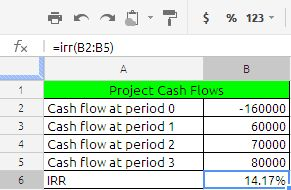Cash flow in period 0, 1 ,2,3, is -160000,60000,70000,80000

to calculate the IRR we have used the formula =irr(B2:B5) the result is 0.141744514349864 i had just formed it to percentage using % in the menu bar to be more understandable to the viewer.

now lets is month rate

If we have taken loan of \$ 100000 for a period of 24 month at the rate of 12% p.a. the EMI works out to \$4707.35 per month. Now we will calculate the IRR to get the rate.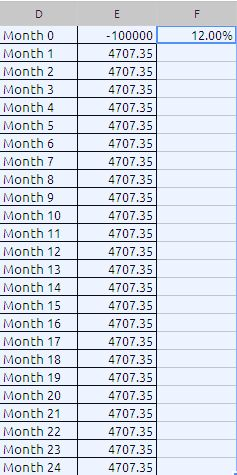The formula in cell F1 is

=irr(E1:E25;10%)*12

we are multiplying the resultant value of irr with 12 to get the annual rate. you may guess value 10%.

the above table is same what we have seen the PMT function. the link to PMT function is

https://anandexcels.wordpress.com/2012/02/04/pmt/

In this way we can calculate IRR for insurance policies, lease, mortgage’s etc

## SUBTOTAL

Subtotal is great function specially when you using filter to your data. The syntax for the function is as follows:

SUBTOTAL(function_code, range_1, range_2, … range_30)
function_code: the subtotal function behave differently depending on the function_code it some time behave like counter,minimum, maximum, sum, average etc. depending on the data range. The complete code list is as follows: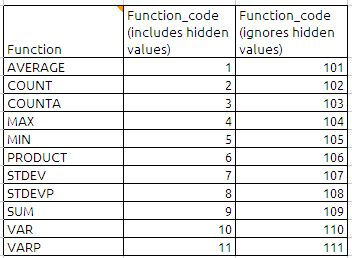ex: if we selected the function_code as 9 then we will get the total of the given range including hidden values. where as if we selected the 109 the function ignores the hidden values in the range. this i will explain in the subsequent paras

range_1,range_2,…..range_30: A collection containing the values to be evaluated. data is a collection containing number, a string  or date/time values or. All values should be of the same type.

Now lets see subtotal function with an example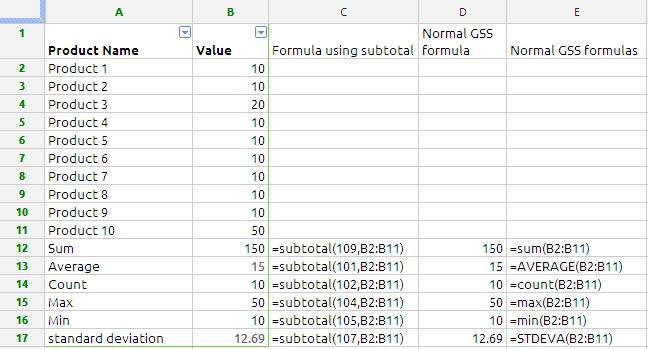The data is from cell A1 to B11, we have formula using subtotal function from B12 to B17 and we have traditional formulas from cell D12 to D17 Google Docs Spread sheets. suppose we filter data and hidden Product1 and Product 2 the data is will be as below: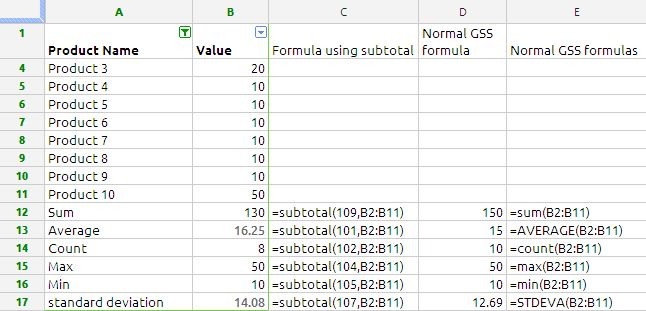the beauty of the Subtotal function is can be clearly seen in the above example. the subtotal formulas are working perfectly and our traditional formula have failed to give correct answers because the traditional formulas are taking the hidden ranges also.

## FREQUENCY

The FREQUENCY function returns an array of how often data values occur within a range of interval values.

FREQUENCY(data, classes)
data:  A collection containing the values to be evaluated. data is a collection containing number or date/time values. All values should be of the same type.

classes:  A collection containing the classes (interval values). interval-values is a collection containing number or date/time values. All values should be of the same type as the values in the data-values collection. Usage Notes

FREQUENCY determines the number of values in data that fall within each interval. The classes (interval array) is easiest to understand if it is arranged in ascending order. The first frequency will be the count of those values that are less than or equal to the lowest interval value. All other frequency values, except the last, will be the count of those values that are greater than the immediately lower interval value and less than or equal to the current interval value. The final frequency value will be the count of those data values that are greater than the largest interval value.

Note : Character sticks of Frequency function
1) Classes are the upper values for each category, and classes are the values to count.
2) FREQUENCY ignores empty cells and text.
3) FREQUENCY function always returns one more category than you give it so that it can  catch any values above the last category.
4) it always delivers a vertical array. So if we really need a horizontal array, we have to use TRANSPOSE function to convert the vertical array to a horizontal array.
5) If there are duplicate classes, the duplicates will get a count of zero (this aspect is utilized when you create formulas for unique counts).
6) since Frequency function happens to be array function google docs spread sheet, we do not need  to enter it with any special key combination.

Now lets see some example with Frequency function.
the formula in cell B2  is
=FREQUENCY(J1:J30,A2:A5)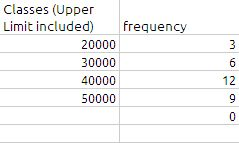The value in cell B6 will come automatically even through there is no range is specified because the formula will include any value above 50000 in this range

If we want to table horizontal the formula will be modified as under: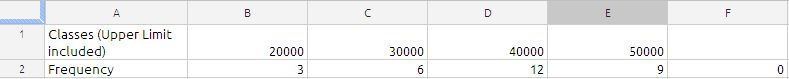the formula in cell B3 is
=TRANSPOSE(FREQUENCY(J1:J30,B1:E1))

Since Frequency function ignore the duplicate. we can utilize this for counting unique value in the data range. The formula for counting the unique value in the range is

=ArrayFormula(sum(FREQUENCY(J1:J30,J1:J30)>0))
In the formula the secret is data and classes as same.

the result will be 21

Sample Data is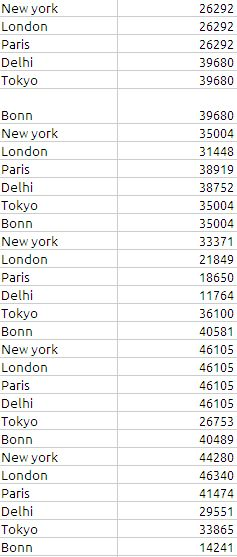## FILTER

The filter function is unique to Google Spread sheets, without this function extracting a duplicate records from a data table will be a very big arrayformula. The power of filter function is in its usage. we will try to understand the basic usage of this function.

The Syntax of Filter function is as follows
FILTER(sourceArray, arrayCondition_1, arrayCondition_2, …, arrayCondition_30)

Explanation given in the help file:

Returns a filtered version of the given source array, where only certain rows or columns have been included. Each condition should be either a 1-dimensional range of boolean values, or else an array-formula expression which evaluates to a 1-dimensional array of booleans. If the conditions evaluate to a column array, then only the rows from the source array corresponding to the true values of the condition array will be returned. Likewise, if the conditions evaluate to a row array, then only the columns of the source array corresponding to the true values in the condition will be returned. If there are multiple conditions, then all must be true for the corresponding values in the source array to be returned.

SourceArray:
The sourcearray is nothing but data source in which we want to apply certain conditions to extract the required results.

arraycondition_1, arrayCondition_2, …, arrayCondition_30 :
We can write the upto 30 conditions to filter the required results.

now lets try to understand the function with an example.

to filter the data we can do it in two ways

(1) Using filter icon  in the menu bar

Adding a filter onto a set of data can help us quickly narrow down the data set to find the data we need. By selecting a data set, we can filter and sort amongst many rows at once. suppose if we want to filter the data table containing the value “Pencil”in the column C

Step 1:
select the Filter icon in the menu bar as shown in the picture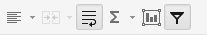Step 2:
by selecting the pencil in the drop down menu as shown the picture below and press OK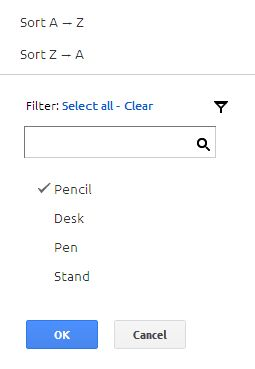The filtered data will be look like this: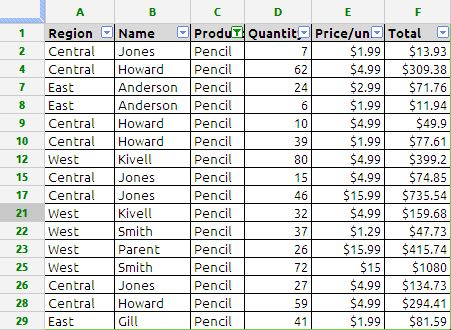The same results can be obtained using filter function in google docs spread sheet by using the following formula
=filter(A2:F30;C2:C30=”Pencil”)

Note: the formula should be entered in the after column F

we can further narrow down the results such as
if we want to filter the data where region is equal to West and Product is equal to Pencil the formula will be
=filter(A2:F30;A2:A30=”West”;C2:C30=”Pencil”)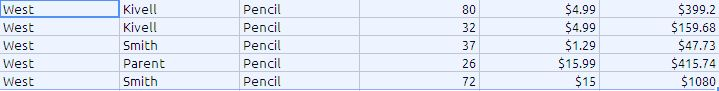in the above formula the function is extracting the data using something like AND condition i.e. the data is shown as if the cell A2:A30 is West and cell C2:C30 is Pencil.

Similarly we can also evaluate the same formula for OR conditions. Suppose we want to extract data which full fills the following conditions:
Column A = West or East
Column C = Pencil

the formula will be
=filter(A2:F30;(A2:A30=”West”)+(A2:A30=”East”);C2:C30=”Pencil”) the results will be as shown below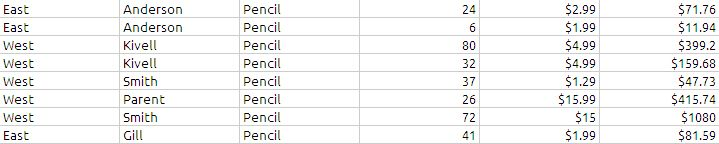The filter function can also be used for single condition or multiple conditional sum
=sum(filter(F2:F30;(A2:A30=”West”)+(A2:A30=”East”);C2:C30=”Pencil”))
it will give the sum of 71.76+11.94+399.2+159.68+47.73+415.74+1080+81.59 which is noting but total of last column of the above picture.

The data table used in this example: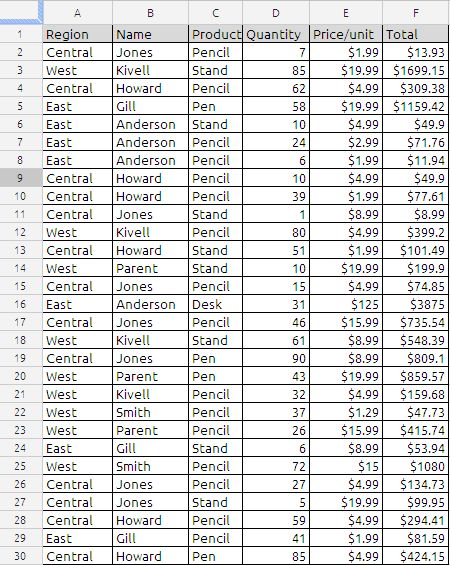## Extracting unique records from two columns

I have two columns:

A              B
Mario       Alen
Dario       Dario
Mario       Alen
Marko    Marko

I want in column C get only unique names from A and B
If I use =UNIQUE this will again create C and D columns, but I need it all in one column. How to do it?

C would look like this:

C
Mario
Dario
Marko
Alen

Solution:
If we try to do this using excel it will ugly big array formula, but the same can be achieved in google docs spread sheets without much fuss and very easy to understand.

I have tried with various formula but i could able to figure it out. Adam Lusk  Top Contributor in the Forum has came up with the following solution.
=ArrayFormula(UNIQUE(TRANSPOSE(SPLIT(CONCATENATE(A:A&CHAR(9);B:B&CHAR(9));CHAR(9)))))

now let us breakup the formula and understand the logic in the solution
Step 1:
=ArrayFormula(CONCATENATE(A:A&CHAR(9);B:B&CHAR(9))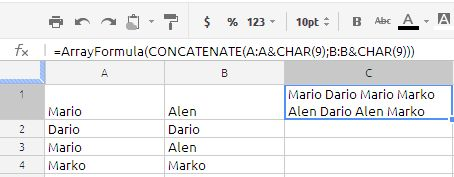the whole data in cell A1 to B4 has been converted into text in the single cell separated by special character char(9) which is nothing but horizontal tabulation.

Step 2:
=ArrayFormula(SPLIT(CONCATENATE(A:A&CHAR(9);B:B&CHAR(9));CHAR(9)))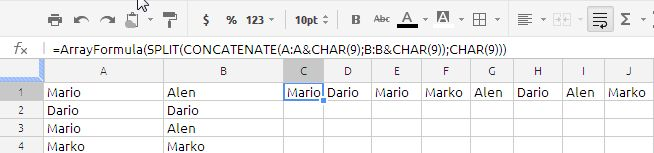in the above picture we have divided the single cell text to multiple cells using split function.

Step 3:
=ArrayFormula(TRANSPOSE(SPLIT(CONCATENATE(A:A&CHAR(9);B:B&CHAR(9));CHAR(9))))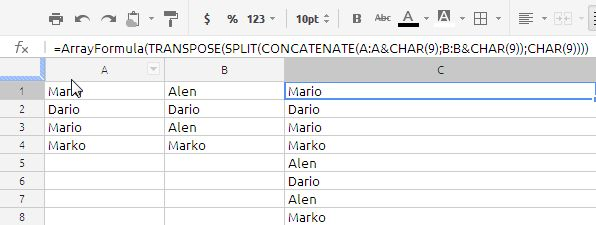we have transposed the horizontal array into vertical array by using the Transpose function. the result will as show in the above picture.

Step 4:
=ArrayFormula(UNIQUE(TRANSPOSE(SPLIT(CONCATENATE(A:A&CHAR(9);B:B&CHAR(9));CHAR(9)))))in the final step we will get required results using the unique function in Google doc.

i would like thank Mr Adam Lusk for the contribution

## SPARKLINE CHARTS

A sparkline chart is data-intense, design-simple, cell-sized graphics

Syntax of the function is as follows:

SPARKLINE(data, [options])

data: the range of cells or array of data to plot on the chart

options: A range or array of optional settings with their associated values used to

customize the chart

Options:
A) charttype: the type of chart to plot (default is “line”)

1. Use “line” for a line chart

2. Use “bar” for a stacked bar chart

B) For line charts only:

1. xmin: sets the minimum value along the horizontal axis; any number is a valid value

2. xmax: sets the maximum value along the horizontal axis; any number is a valid value

3. ymin: sets the minimum value along the vertical axis; any number is a valid value

4. ymax: sets the maximum value along the vertical axis; any number is a valid value

C) For bar charts only:

1. max: sets the maximum value along the horizontal axis; any number is a valid value

Notes on sparkline syntax

• For line charts, “data” can either be a 1-dimensional array of values or a 2-dimensional array containing values and spacing along the horizontal axis.
• For bar charts, only one row or column of data can be plotted.
• The “text color” and “text background color” buttons in the spreadsheet toolbar can be used to set the color and background color for line charts.
• Each sparkline function only accepts a few hundred data points. If a broken image icon is shown in the cell, try reducing the number of data points plotted on the chart and try again.

Lets see some examples: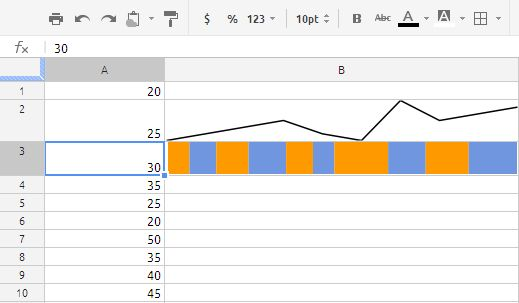in cells A1:A10 we have random number between 20 to 50

the formula in cell B2 is
=SPARKLINE(A1:A10)
and the formula in cell B3 is
=SPARKLINE(A1:A10,{“charttype”,”bar”})

From the above it is understood that if we leave the options we will get only line chart,
if we want to prepare the barchart we have to use the options argument.

=SPARKLINE(A2:E2, A4:B5)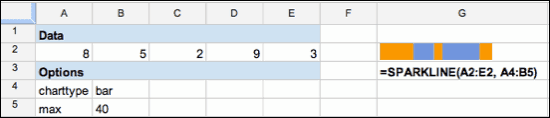in the above example we have to observe one important thing:
the options are in two row height and two column width. It has to be like that otherwise the function does not work because if you observe the formula without referring to cells it will be like this

=SPARKLINE(A2:E2,{“charttype”,”bar”;“ymax”,10})

observe semi-colon between “bar” and “ymax” here semi-colon means it is two dimensional array you can add as many options as require by following the above principle.

Plot the price of a stock over a 360-day period for apple is as follows:

=SPARKLINE(GOOGLEFINANCE(“aapl”,”price”,TODAY()-30,TODAY()))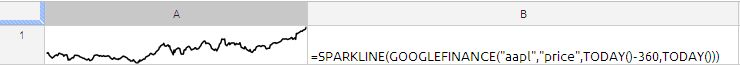Similarly we can generate the Square line charts, Sinwave charts, SawTooth wave charts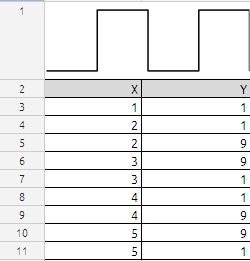formula in cell A1 is

=SPARKLINE(A3:B11, {“charttype”,”line”;”ymax”,10;”ymin”,0} )